# Maharashtra Board Class 11 Economics Solutions Chapter 3 Partition Values

Balbharti Maharashtra State Board Class 11 Economics Solutions Chapter 3 Partition Values Textbook Exercise Questions and Answers.

## Maharashtra State Board Class 11 Economics Solutions Chapter 3 Partition Values

1. Choose the correct option:

Question 1.
Statements that do not apply to Quartiles.
(a) First arrange the values in ascending or descending order.
(b) Observation can be divided into 4 parts.
(c) They are represented as Q1, Q2, and Q3
(d) Q2 is also known as the median.
Options:
(1) a
(2) b and c
(3) a, b and c
(4) None of these
(4) None of these

Question 2.
D7 from the given data.
Data – 4, 5, 6, 7, 8, 9, 10, 11, 12
Options:
(1) 7
(2) 9
(3) 10
(4) 12
(1) 7Question 3.
Statements related to partition values that are correct.
(a) Exact divisions of percentiles into 100 parts gives 99 points
(b) Deciles have total of 9 parts
(c) Quartiles are shown by Q1, Q2, and Q3
(d) Symbolically, Percentiles and Deciles are shown by P and D
Options:
(1) a and c
(2) a and b
(3) a, b and c
(4) a, c and d
(4) a, c and d

2. Identify the correct pairs from the given options: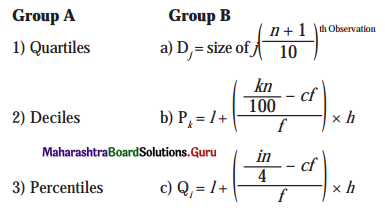Options:
(1) 1-b, 2-c, 3-a
(2) 1-c, 2-a, 3-b
(3) 1-c, 2-b, 3-a
(4) 1-a, 2-b, 3-c
Correct Pair: (2) 1-c, 2-a, 3-b

3. Give economic terms:

Question 1.
Procedure for dividing the data into equal parts.
Partitioning

Question 2.
The value that divides the series into ten equal parts.
Deciles

Question 3.
The value that divides the whole set of observations into four equal parts.
Quartiles

4. Solve the following:

Question 1.
Calculate Q1, D4, and P26 for the following data.
18, 24, 45, 29, 4, 7, 28, 49, 16, 26, 25, 12, 10, 9, 8
Q1 = 9, D4 = 13.6, P26 = 9.16Question 2.
Calculate Q3, D5, and P35 for the given data.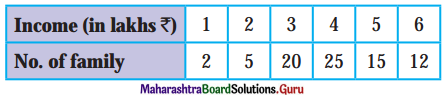Q3 = ₹ 5 Lakhs, D5 = ₹ 4 Lakhs, P35 = ₹ 4 Lakhs

Question 3.
Find out P50 for the following data: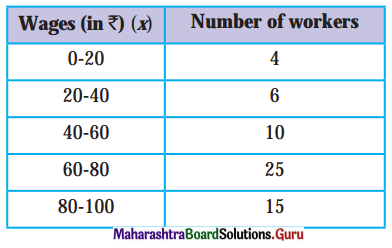P50 = ₹ 68 Wages

Question 4.
Calculate Q3 for the following data: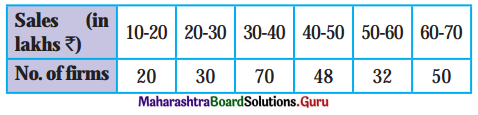Q3 = ₹ 56.09 Lakhs

Question 5.
Calculate D7 for the following data.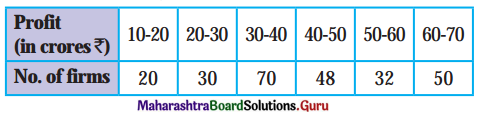D7 = ₹ 52.1875

Question 6.
Calculate P15 for the following data.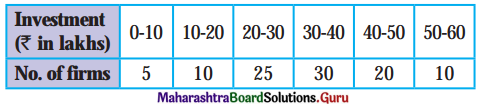P15 = ₹ 20 lakhs5. State with reasons whether you agree or disagree with the following statements:

Question 1.
Partition values have application only in theory but not in practice.
OR
Partition values are not useful in economics.
No, I do not agree with the statement.

• Partition values like quartiles are useful to economists to know the final information related to income, sales, stock data, etc.
• Also, deciles and percentiles help economists to measure in the detail the poverty line, inequality of income, household, wealth, etc.
• Hence, partition values are useful in Economics.

Question 2.
Average can misinterpret the representative value.
Yes, I agree with the statement.

• Average is not based on all observations because it is a positional average and their values are affected by sampling fluctuations.
• Averages give more importance to larger values and less to smaller values.
• Thus, such data can misinterpret the representative value.Question 3.
Median is also known as the second quartile.
Yes, I agree with the statement.

• Median is the middlemost value in the arranged data.
• It is the value that divides the series into two equal parts, so that the number of items above it, is equal to the number of items below it.
• It is not affected by extreme values.
• Median = n/2 and Q2 = 2 (n/4) i.e n/2.
• Therefore, the Median is equal to the second quartile.

6. Answer the following questions on the basis of the given table: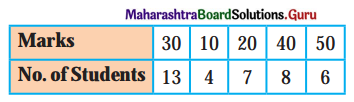Question 1.
Write the formula of Q1 and Q3.
Q1 = size of $$\left(\frac{n+1}{4}\right)^{\text {th }}$$ Observation
Q3 = size of 3 $$\left(\frac{n+1}{4}\right)^{\text {th }}$$ Observation

Question 2.
Find out the cumulative frequency of the last value in the above data.
Cumulative frequency = 38.

Question 3.
Find out the value of ‘n’ in the above data.
n = 38

Question 4.
Find out the median of the above data?
Median = 30.

11th Economics Digest Chapter 3 Partition Values Intext Questions and Answers

Do you know? (Text Book Page No. 14)

Statistics Day: Prof. Prasanta Chandra Mahalanobis, an Indian Statistician was instrumental in formulating India’s strategy for industrialization in the Second Five Year Plan (1956-61) which later came to be known as Mahalanobis Model.

Mahalanobis devised a measure of comparison between two data sets that are known as the Mahalanobis distance. He also devised a statistical method called ‘fractile graphical analysis’ which could be used to compare the socio-economic conditions of different groups of people. In recognition of the notable contributions made by P. C. Mahalanobis in the field of economic planning and statistical development, the Government of India has designated the 29th of June every year, coinciding with his birth anniversary as ‘Statistics Day’, in the category of Special day to be celebrated at the national level.Question 1.
Who formulated India’s strategy for industrialization?
Prof. Prasanta Chandra Mahalanobis, an Indian Statistician formulated India’s strategy for industrialization.

Question 2.
What is the Mahalanobis distance?
A measure of comparison between two data sets is called Mahalanobis distance.

Question 3.
What is fractile graphical analysis?